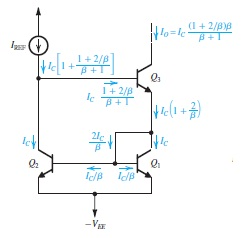#### All Pages

Assignment Help Humanities
##### Reference no: EM131037264

1. For the current-mirror-loaded bipolar differential pair, replacing the simple current-mirror load by the Wilson mirror of Fig. 8.40(a), find the expected systematic input offset voltage. Evaluate VOS for βP,= 50.##### Reference no: EM131037264

For the current-mirror-loaded bipolar differential pair, replacing the simple current-mirror load by the base-current-compensated mirror of Fig. 8.11, find the expected syst

#### Input resistance of the subsequent stage is equal to rid

The differential amplifier in Fig. 9.36(a) is operated with I = 500 μA, with devices for which VA =10 V and β =100. What differential input resistance, output resistance, sh

#### Nmos differential amplifier operates

A current-mirror-loaded NMOS differential amplifier operates with a bias current I of 200 μA. The NMOS transistors are operated at VOV = 0.2 V and the PMOS devices at /VOV/

#### Design a mos differential amplifier

D 9.14 Design a MOS differential amplifier to operate from ±1-V power supplies and dissipate no more than 1 mW in the equilibrium state. The differential voltage gain Ad is

#### Design the mos differential amplifier

D 9.10 Design the MOS differential amplifier of Fig. 9.5 to operate at VOV =0.25 V and to provide a transconductancegm of 1 mA/V. Specify the W/L ratios and the bias current.

#### Amos differential amplifier biased

9.9 AMOS differential amplifier biased with a current source I =200 μA is found to switch currents completely to one side of the pair when a difference signal vid =0.3 V is

#### Maximum possible fractional change

9.8 Use Eq. (9.23) to show that if the term involving v2 id is to be kept to a maximum value of k then the maximum possible fractional change in the transistor current is gi

#### Maximum possible fractional change

9.8 Use Eq. (9.23) to show that if the term involving v2 id is to be kept to a maximum value of k then the maximum possible fractional change in the transistor current is gi

### Write a Review